# QuTiP development notebook: test number state basis with lower cut off¶

Copyright (C) 2013, Paul D. Nation & Robert J. Johansson

When we work with harmonic oscillator operators in the number state representation we always need to truncate the number of basis states at a finite number. Usually we pick an upper limit for the number state and use all the number states below this cut off. However, in some problems it can also be useful with a number basis representation that does not start with the zero number state, but rather at some lower cut off. This notebook demonstrates how to do this in QuTiP using the optional offset argument to oscillator operator functions.

In :
%pylab inline

Populating the interactive namespace from numpy and matplotlib

In :
from qutip import *


## Test operators with offset¶

In :
def steadystate_fock_distribution(N, offset):
"""
Solve for the steady state of a driven dissipative cavity, given
the upper (N) and lower (offset) cut offs for the number states in
in the basis. Calculate the average photon number in the steady
state and plot the fock state distribution.
"""
N = N - offset
Omega = 1.0 * 2 * pi
kappa = 0.9

a = destroy(N, offset)
n = num(N, offset)
H = Omega * (a + a.dag())
c_ops = [sqrt(kappa) * a]

title = "Steady state avg. photon number = %.2f" % expect(rho_ss, n)

fig, ax = fock_distribution(rho_ss, offset=offset, unit_y_range=False, title=title)

return fig, ax

In :
steadystate_fock_distribution(250, 0);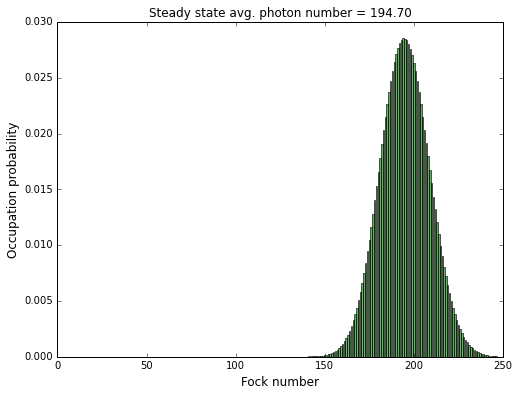In :
steadystate_fock_distribution(250, 150);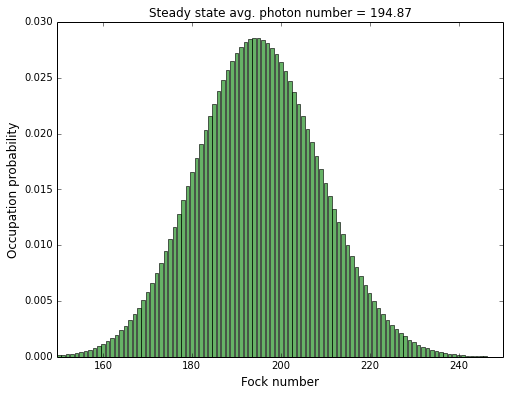## Test states with offset¶

In :
%pylab inline

Populating the interactive namespace from numpy and matplotlib

In :
from qutip import *


### Fock states¶

In :
N = 10
offset = 0

n = num(N, offset=offset)
psi = (basis(N, 2, offset=offset) + basis(N, 4, offset=offset) + basis(N, 6, offset=offset)).unit()

fock_distribution(psi, offset=offset, unit_y_range=False, figsize=(4,3))

expect(n, psi)

Out:
4.000000000000001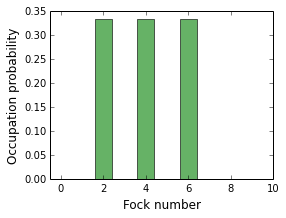In :
N = 5
offset = 2

n = num(N, offset=offset)
psi = (basis(N, 2, offset=offset) + basis(N, 4, offset=offset) + basis(N, 6, offset=offset)).unit()

fock_distribution(psi, offset=offset, unit_y_range=False, figsize=(4,3))

expect(n, psi)

Out:
4.000000000000001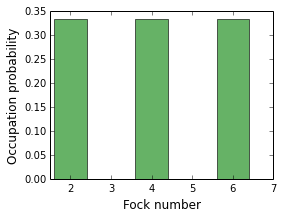### Coherent states¶

In :
N = 75
offset = 0

n = num(N, offset=offset)
psi = coherent(N, 6.5, offset=offset)

fock_distribution(psi, offset=offset, unit_y_range=False, figsize=(4,3))

expect(n, psi)

Out:
42.24999120237548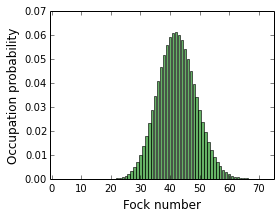In :
N = 50
offset = 20

n = num(N, offset=offset)
psi = coherent(N, 6.5, offset=offset)

fock_distribution(psi, offset=offset, unit_y_range=False, figsize=(4,3))

expect(n, psi)

Out:
42.244955490644436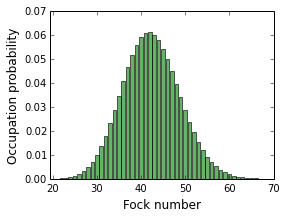## Software versions¶

In :
from qutip.ipynbtools import version_table; version_table()

Out:
SoftwareVersion
Cython0.19.1
QuTiP2.3.0.dev-15c5f51
SciPy0.13.0.dev-7c6d92e
Python3.3.1 (default, Apr 17 2013, 22:30:32) [GCC 4.7.3]
Numpy1.8.0.dev-b307a8a
matplotlib1.4.x
IPython2.0.0-dev
OSposix [linux]
Fri Aug 30 15:46:41 2013 JST